# NMath User's Guide

17.7 Symmetric Banded Matrices (.NET, C#, CSharp, VB, Visual Basic, F#)

A symmetric banded matrix is a symmetric matrix (Section 17.3) that has all its non-zero entries near the diagonal. Entries farther away from the diagonal than the half bandwidth are defined to be zero. That is, ifis the half bandwidth, then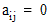whenever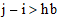or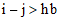. For example, this is a 5 x 5 symmetric banded matrix with a half bandwidth of 1: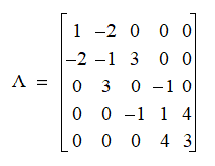Symmetric banded matrices often arise in one-dimensional finite element problems.

NMath provides symmetric banded matrix classes for single- and double-precision floating point numbers. The classnames are and . Hermitian banded matrices are a generalization of symmetric banded matrices for complex types (Section 17.8).

For efficiency, the lower triangular part of the matrix and zero elements outside the bandwidth are not stored. Instead, matrix values are stored in a vector column by column. Blank entries are inserted in the data vector so that the each column takes up the same number of elements,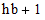, in the vector. For example, the following 8 x 8 matrix with a half bandwidth of 2:is stored in a data vector as:

```v = [x   x   a00
```
```     x   a01 a11
```
```     a02 a12 a22
```
```     a13 a23 a33
```
```     a24 a34 a44
```
```     a35 a45 a55
```
```     a46 a56 a66
```
```     a57 a67 a77 ]
```

where x denotes an unused location.

Top

Top